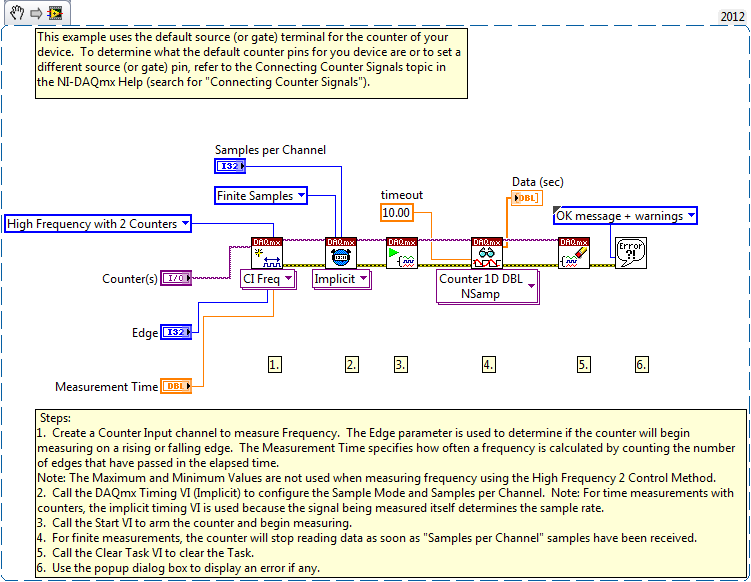# Example Code

## Products and Environment

This section reflects the products and operating system used to create the example.

### Hardware

• Data Acquisition (DAQ)

• NI DAQmx

## Code and Documents

### Attachment

Overview

These VIs demonstrate three different methods for making frequency measurements.
The three methods for making frequency measurements are:
Method 1: Period Measurement
Method 2: Pulse Count over a Known Time
Method 3: Time Measurement of a Known Number of cycles

Description

Method 1: The simplest method is period measurement. You can measure the period by counting the rising or falling edges of a known source frequency between the two consecutive rising or falling edges of the unknown frequency. By taking the frequency of the known source and dividing by the count, you can calculate the period of the unknown signal. This type of measurement is suitable for low frequency measurement.

Method 2: Method 1 works well as long as the frequency of the signal to be measured is significantly slower than the known source frequency. As the frequency of the signal to be measured increases to approach the frequency of the source frequency, measurement error increases. To improve the accuracy of the frequency measurement for higher unknown frequency, we can use this second measurement method, count number of pulses in known time. In this configuration, the counter will count number of unknown high frequency during a period of known signal. The frequency can be calculated by multiplying the count by the frequency of the unknown signal.
By using two counters, you can get more accurate frequency measurement for higher frequencies. The larger the period of the known signal used to gate the counting, the smaller the measurement error.

Method 3: The inverse period measurement method is suitable for lower frequency measurements while the second measurement method can achieve higher accuracy with higher frequency signals. However, if your application involves measuring a pulse width modulated signal and the span of the frequency range covers both low and high frequencies, then this third measurement method can be considered.

Similar to measurement method 2, count number of pulses in known time, this measurement method also uses two counters. The first counter is used to divide down the frequency of the signal to be measured; then the second counter is used to measure the period of the divide down frequency. The actual frequency can be calculated by multiplying the resulting frequency measurement by the divide down value. With this frequency method, the larger the divide down value, the slower the resulting frequency, and more accurate the measurement result.

Requirements

LabVIEW 2012 (or compatible)

DAQmx 9.5.5 (or compatible)

Steps to Implement or Execute Code

1. Set Channel Parameters and Timing Parameters
2. Run VI

Method 1Method 2Method 3**This document has been updated to meet the current required format for the NI Code Exchange.**

Example code from the Example Code Exchange in the NI Community is licensed with the MIT license.